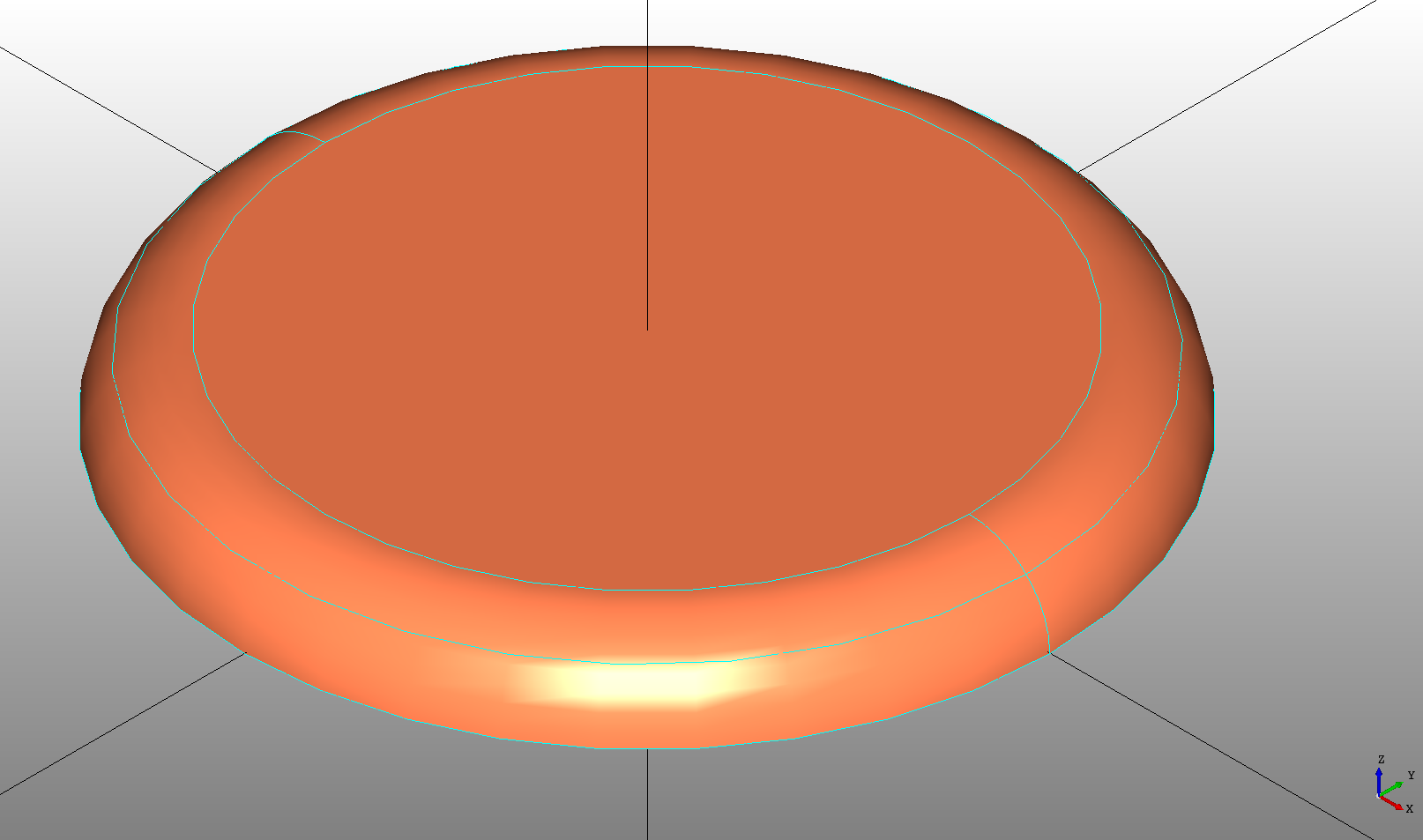## Primitive Shapes

DeclaraCAD supports the following primitive shapes to help start modeling.

## Box

The `Box` declaration creates a cube or extruded rectangle relative at the bottom left corner from `dx`, `dy`, and `dz` dimensions.

Note: The box can be centered by setting the `position = (-dx/2, -dy/2, -dz/2)`.

``````# Created in DeclaraCAD

enamldef Assembly(Part):
Box:
color = 'coral'
transparency = 0.8
dx = 5
dy = 6
dz = 10
``````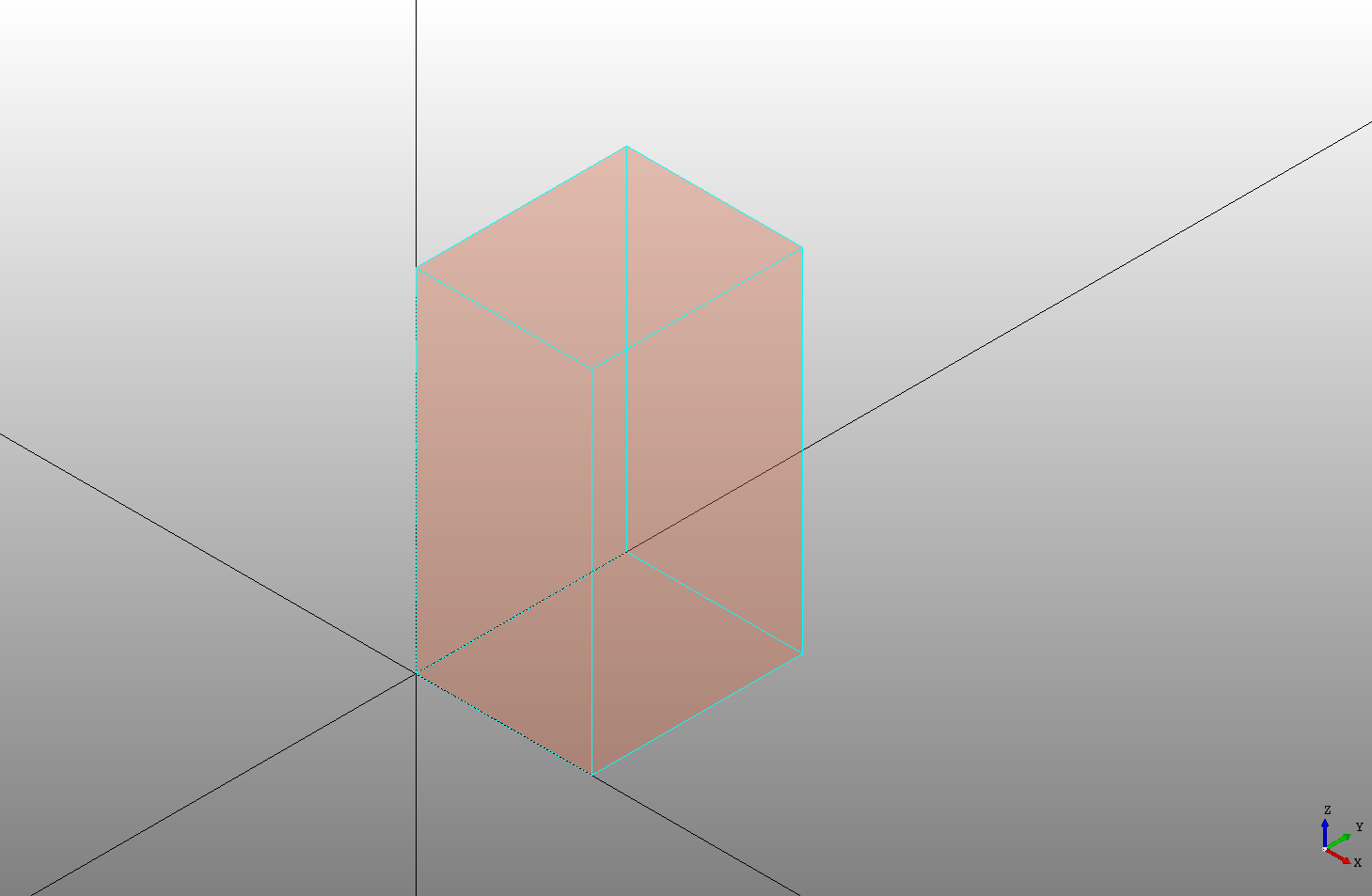## Wedge

The `Wedge` declaration creates a wedge or extruded triangle. The `dx`, `dy` and `dz` define the triangle and it is positioned at the bottom left corner and may be centered in the same way as the box.

``````# Created in DeclaraCAD

enamldef Assembly(Part):
Wedge:
color = 'coral'
transparency = 0.5
dx = 4
dy = 1
dz = 2
``````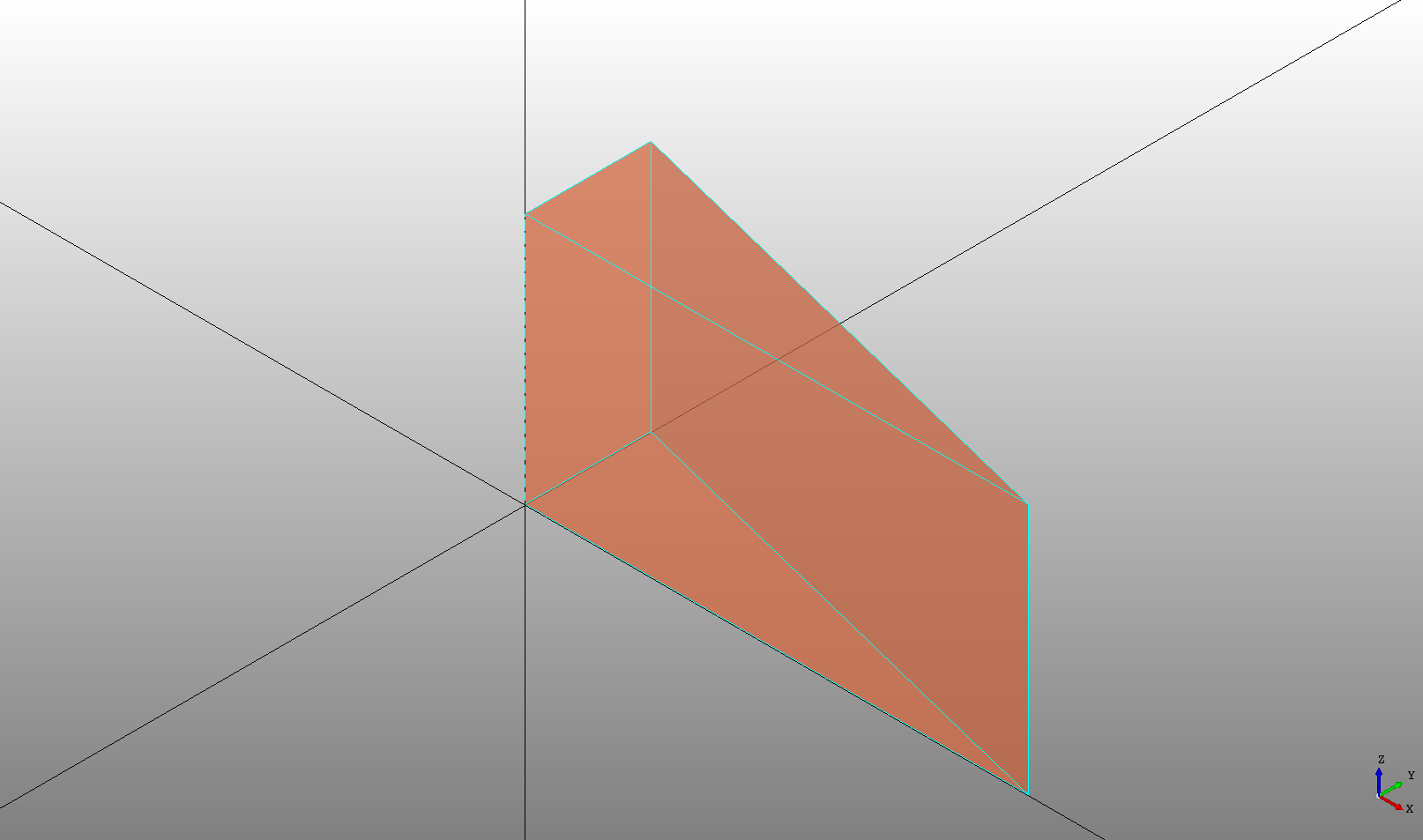The `itx` attribute can be set to adjust where the edge starts, by default it is at 0.

``````# Created in DeclaraCAD

enamldef Assembly(Part):
Wedge:
color = 'coral'
transparency = 0.5
dx = 4
dy = 1
dz = 2
itx = dx/2
``````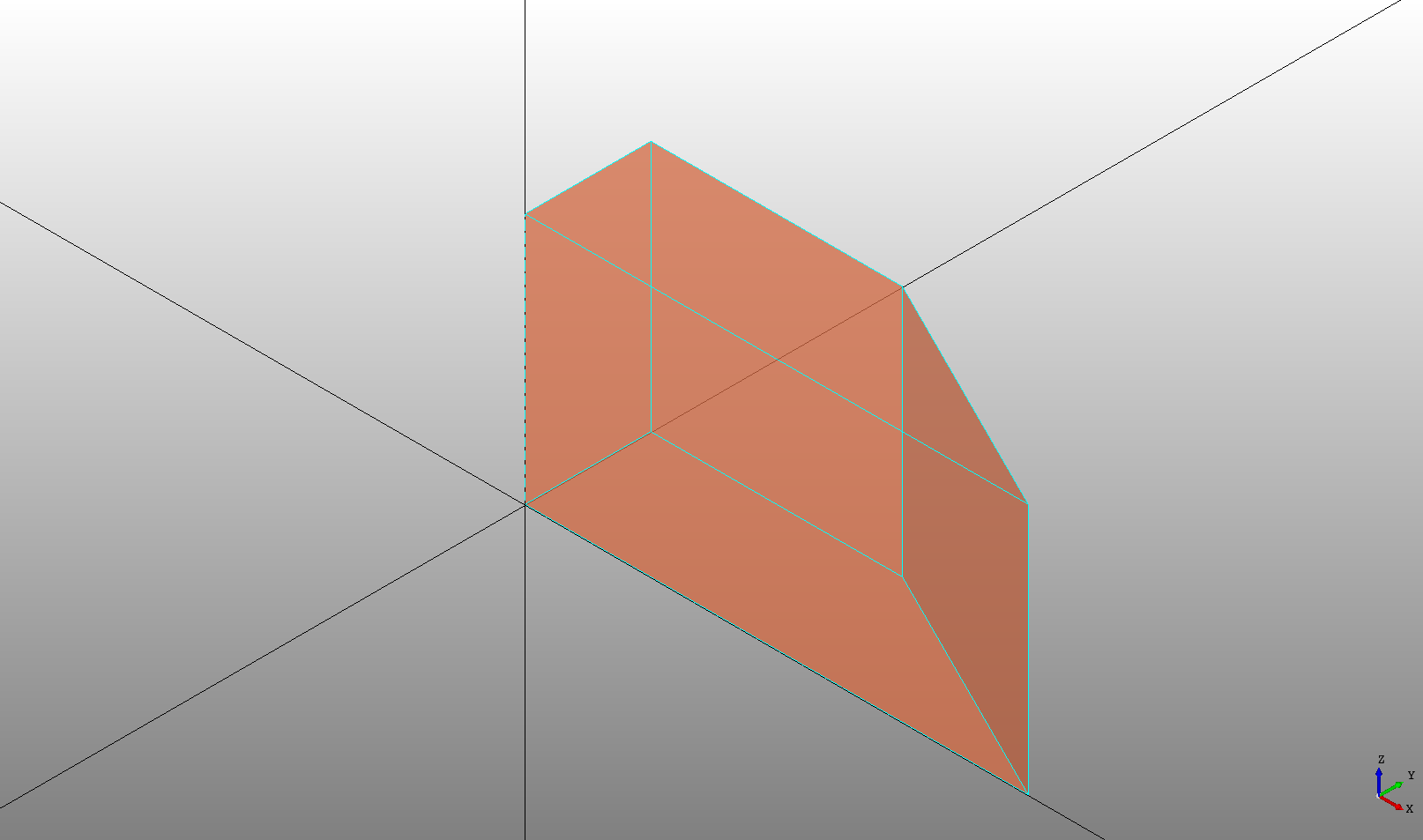## Cylinder

The `Cylinder` declaration creates a cylinder from a `radius` and `height` in the given `direction`. The cylinder is starts at the `position` and extends in the `direction` by the `height`.

``````# Created in DeclaraCAD

enamldef Assembly(Part):
Cylinder:
color = 'coral'
transparency = 0.5
height = 5
``````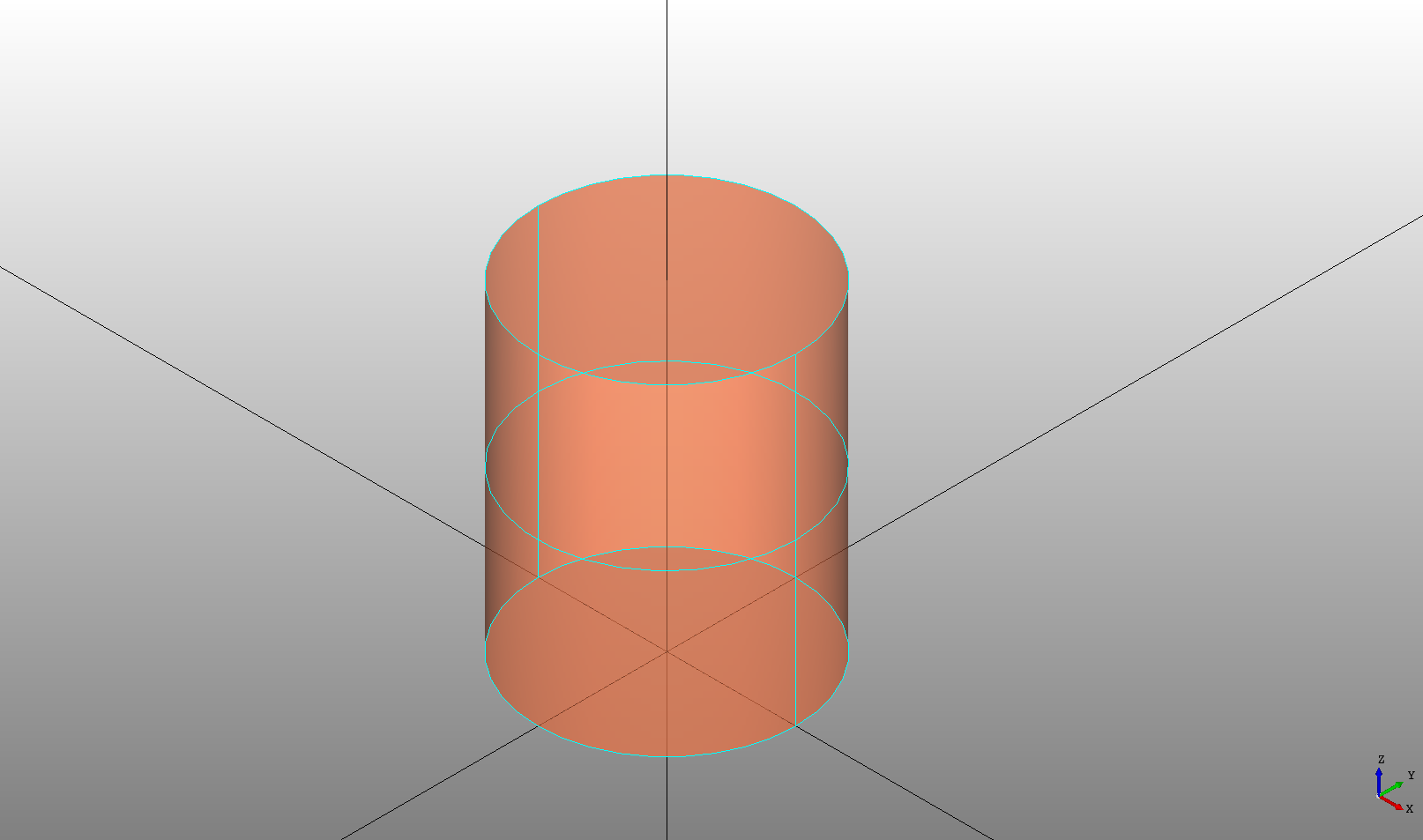An `angle` can be specified in radians to limit the angle of revolution.

``````# Created in DeclaraCAD
from math import pi

enamldef Assembly(Part):
Cylinder:
color = 'coral'
transparency = 0.5
height = 5
angle = pi/2
``````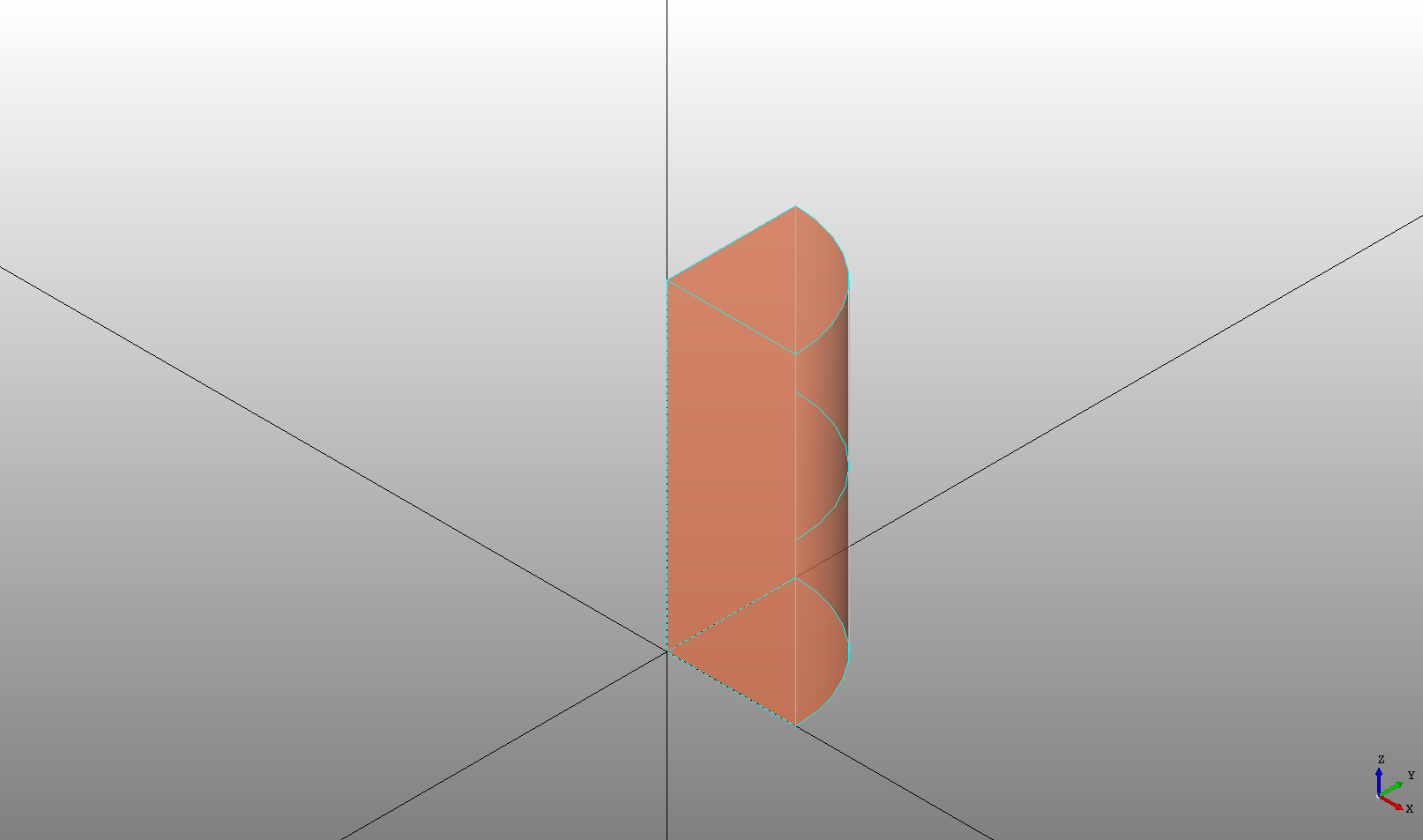## Tube

The `Tube` is a shortcut for a hollowed out cylinder. The parameters are the same but it takes a `radius2`. The smaller of `radius` and `radius2` will be used as the inner radius cut out of the tube. If either `radius` or `radius2` is zero it will create a cylinder.

``````# Created in DeclaraCAD

enamldef Assembly(Part):
Tube:
height = 6
Tube:
position = (10, 0)
height = 6
Tube:
position = (0, 10)
height = 6

Tube:
position = (-10, 0)
height = 6
``````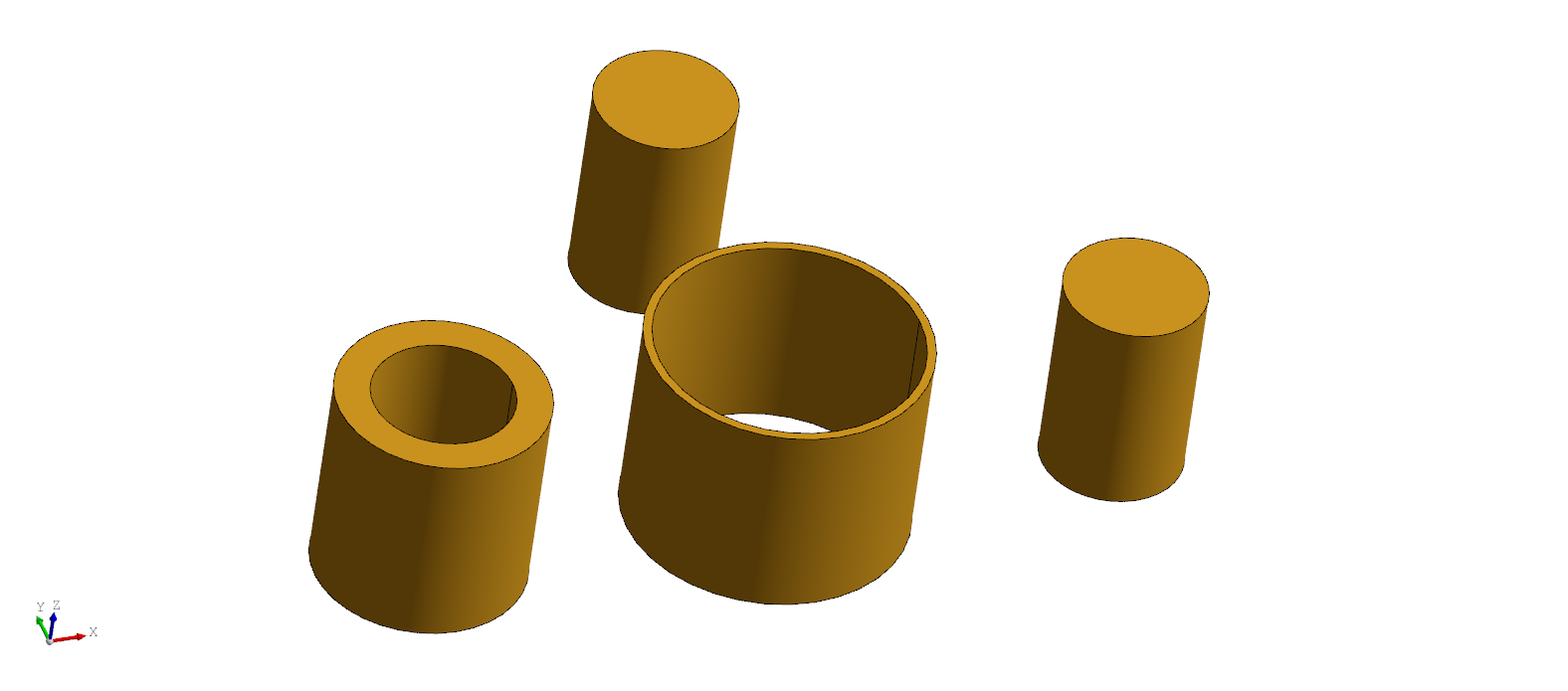## Cone

The `Cone` declaration creates a tapered cylinder. It is defined the same as a cylinder with a `radius` and `height` and may also be limited by an `angle`.

``````# Created in DeclaraCAD
from math import pi

enamldef Assembly(Part):
Cone:
color = 'coral'
height = 5
``````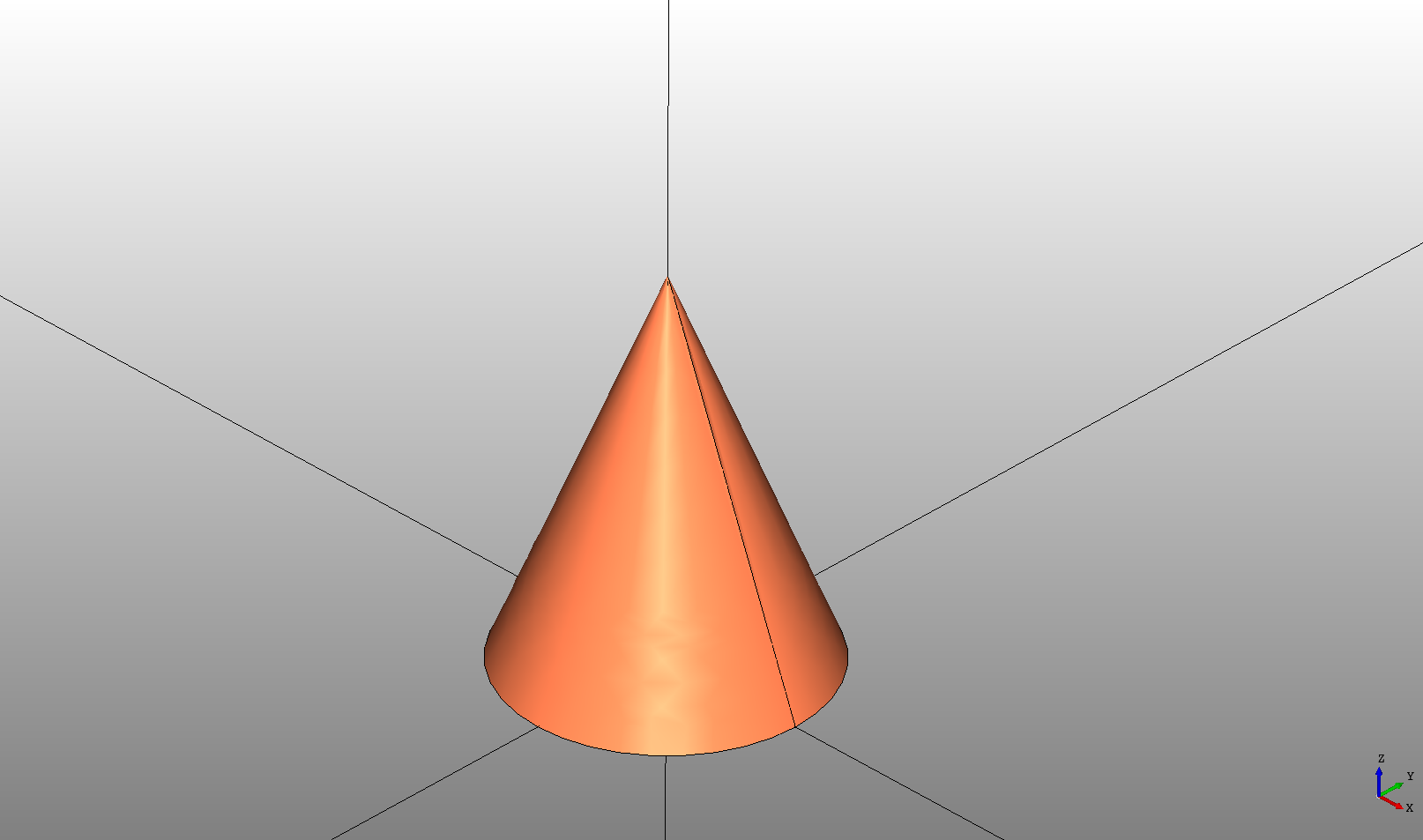The `radius2` attribute may be given to limit the radius where it stops.

``````# Created in DeclaraCAD
from math import pi

enamldef Assembly(Part):
Cone:
color = 'coral'
height = 5
``````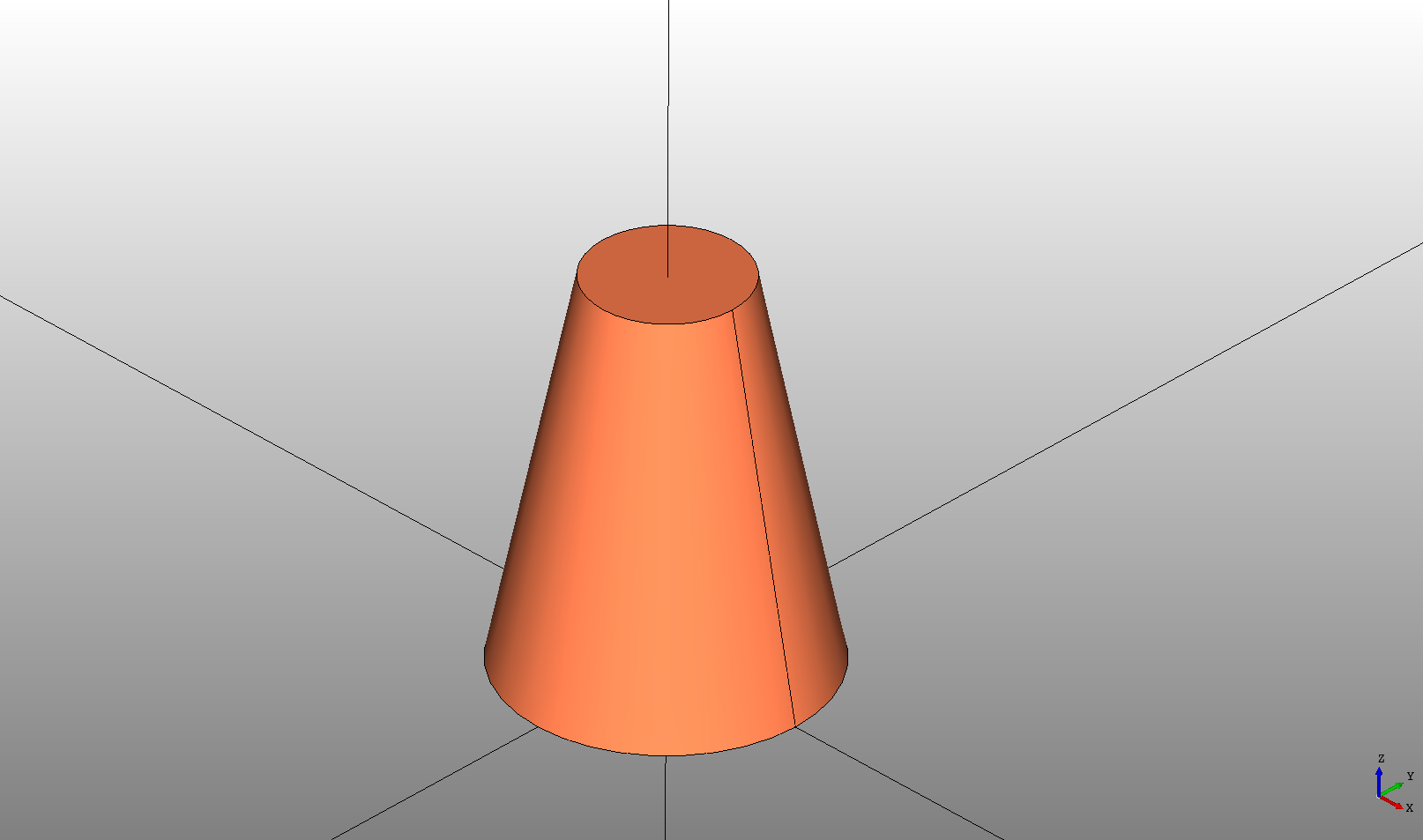## Sphere

The `Sphere` declaration creates a ball or sphere centered at the given position. The `radius` attribute defines the size.

``````# Created in DeclaraCAD
from math import pi

enamldef Assembly(Part):
Sphere:  s1:
color = 'coral'
transparency = 0.5
``````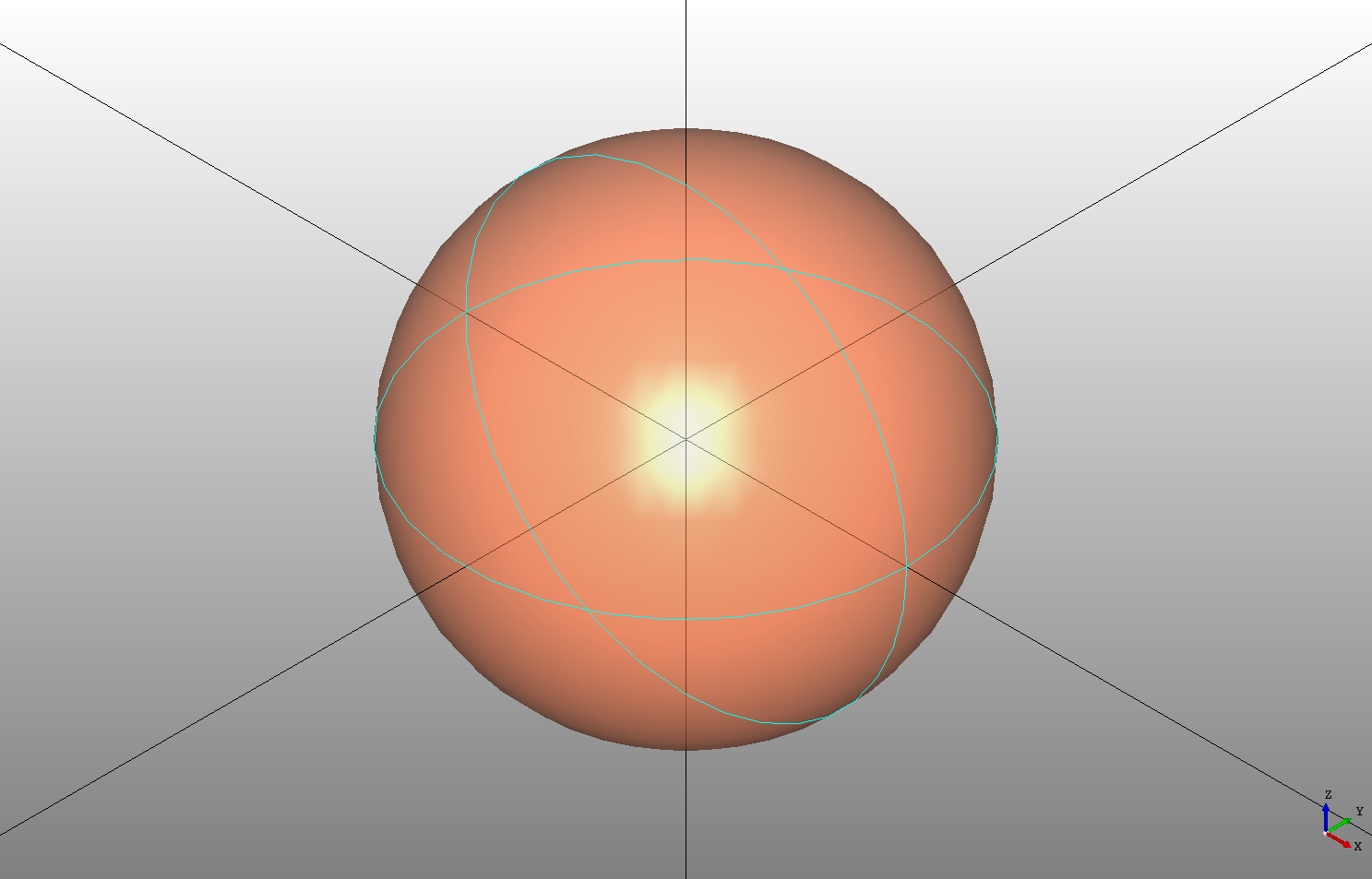The sphere can be reduced to a section by setting `angle`, `angle2`, and `angle3` which limit the sphere in the u and v ranges.

The `angle` can range from `0` to `2*pi` to limit the sphere around the `direction` axis. For example `angle = pi` (shown below) will create a half sphere, `angle = pi/2` will create a quarter sphere.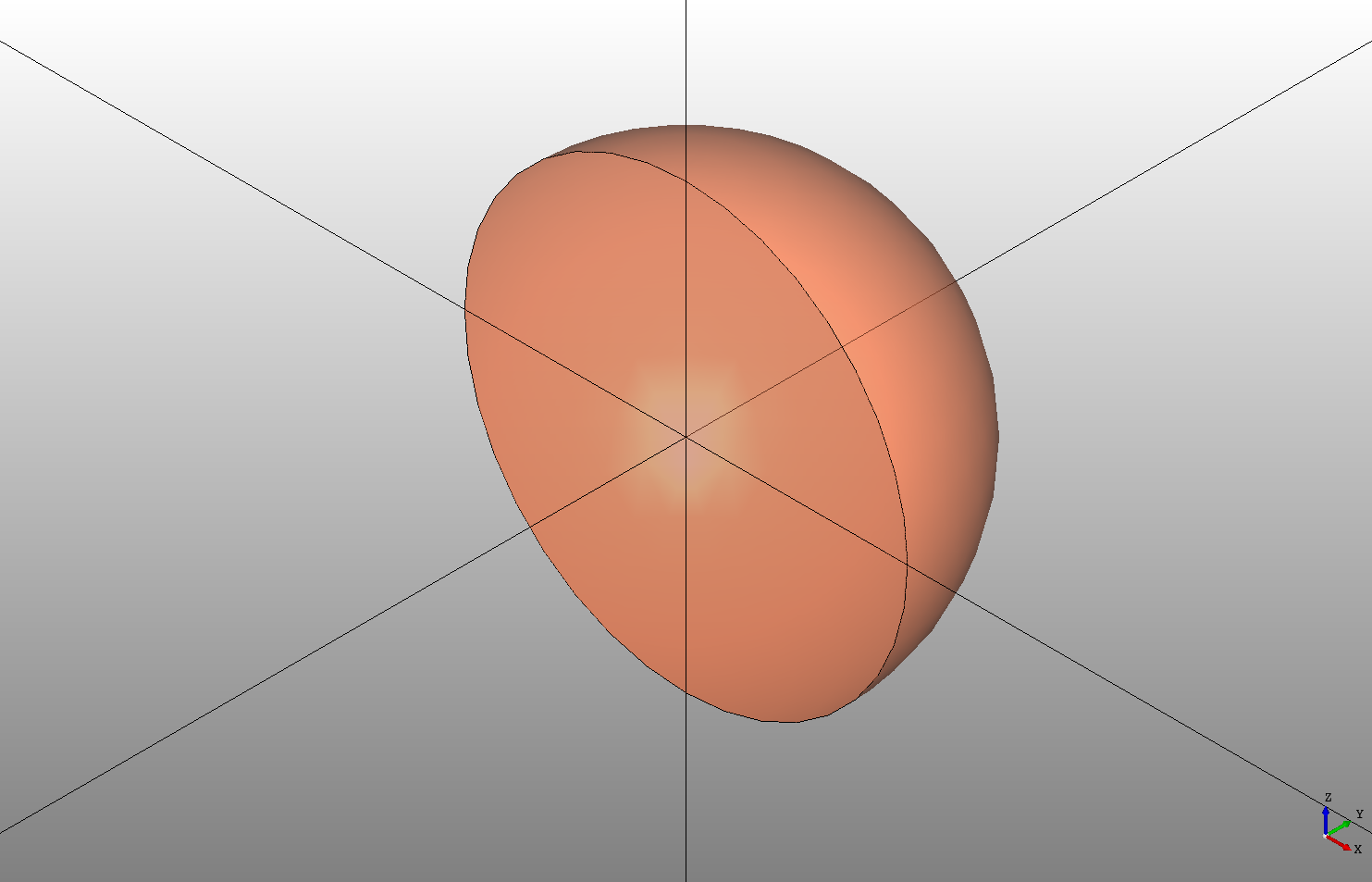The other two `angle2` and `angle3` range from `-pi/2` to `pi/2` and limit the sphere in the opposite sweep direction.

``````# Created in DeclaraCAD
from math import pi

enamldef Assembly(Part):
Sphere:  s1:
color = 'coral'
transparency = 0.5
angle2 = -pi/4
angle3 = pi/4
``````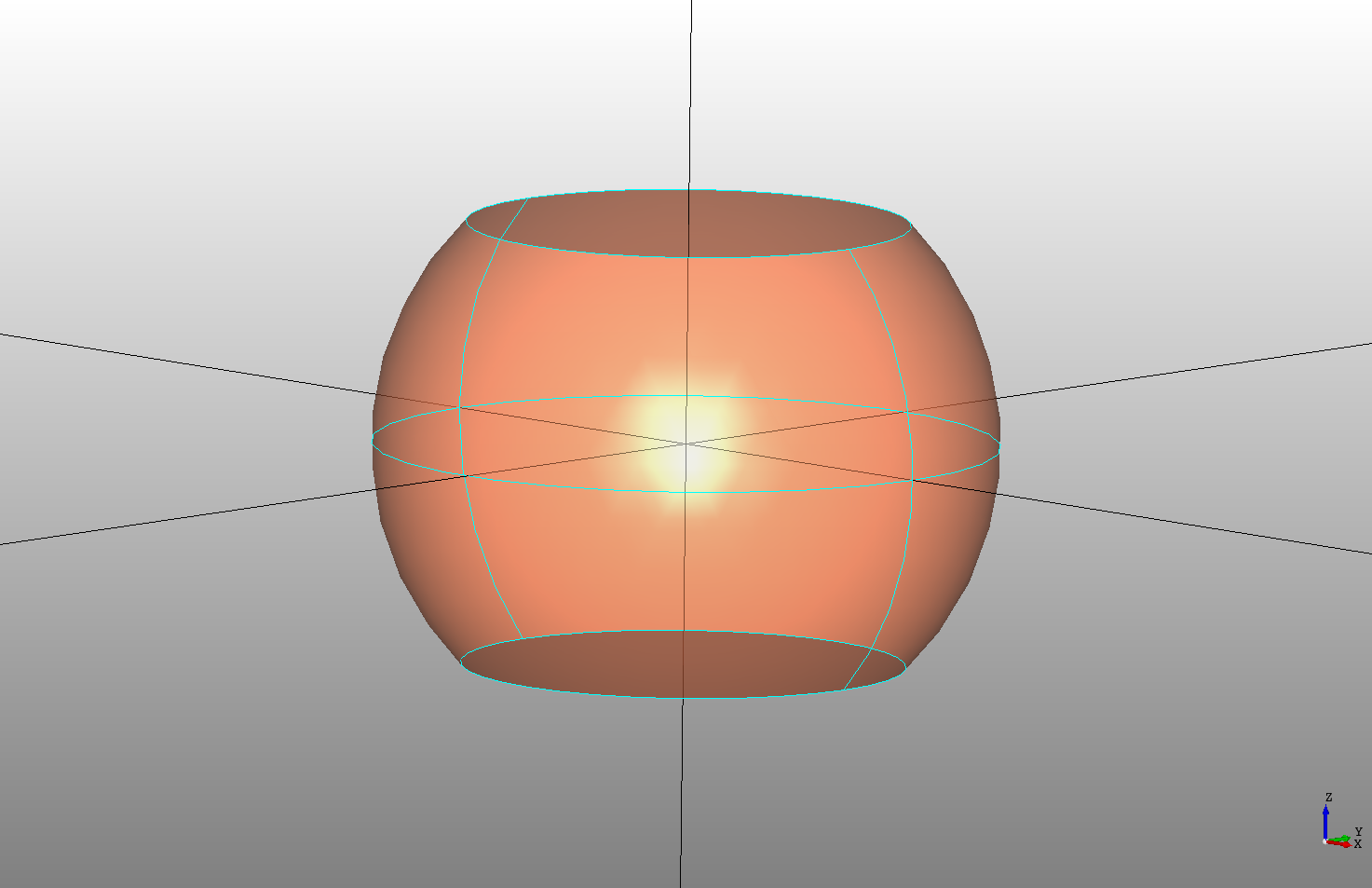## Torus

The `Torus` declaration creates a circle revolved around a radius or a "ring" shape. Set the `radius` attribute for the revolve radius and the `radius2` for the profile radius.

``````# Created in DeclaraCAD
from math import pi

enamldef Assembly(Part):
Torus:  s1:
color = 'coral'
``````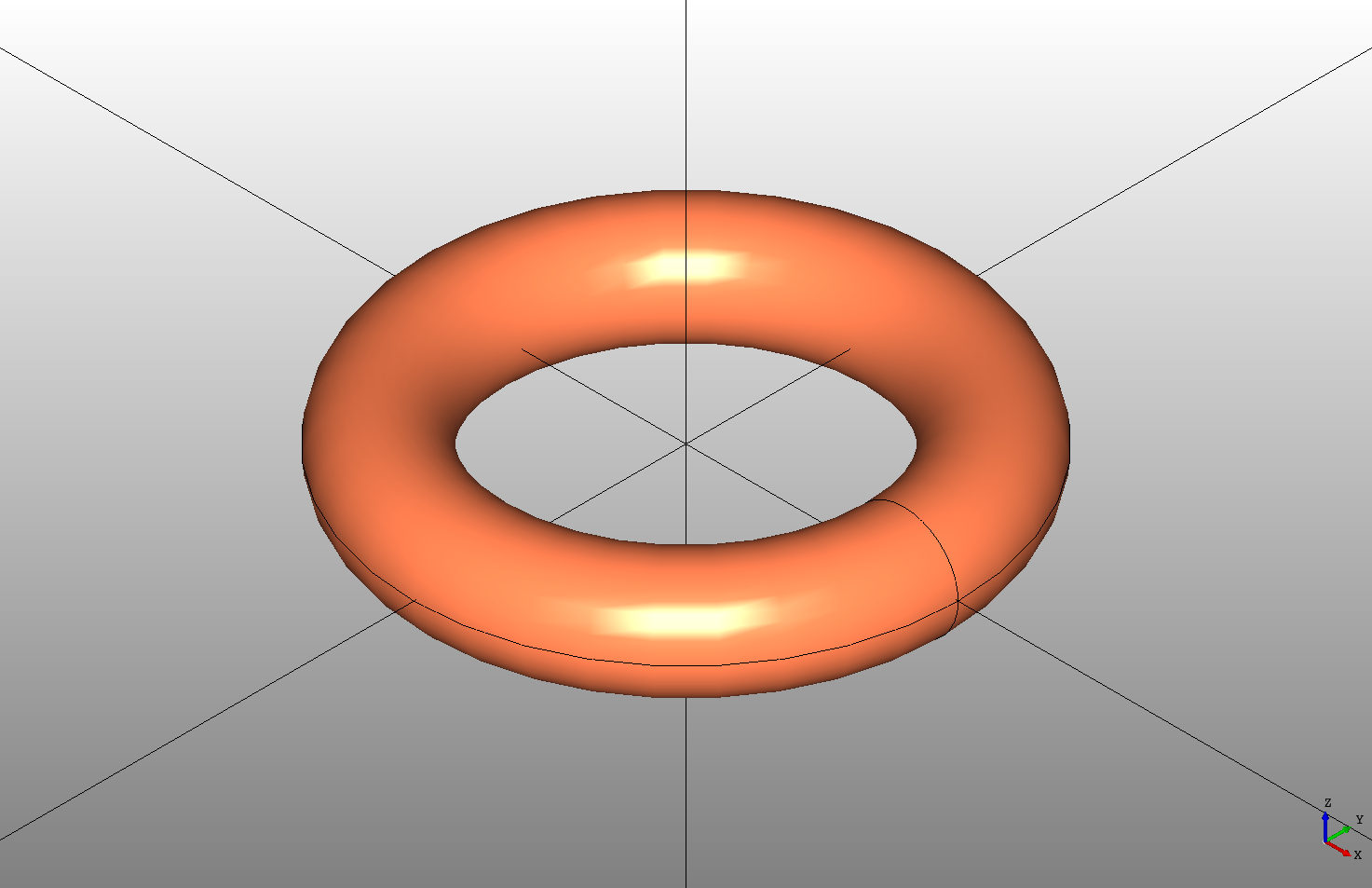The torus can also be limited by the `angle` in radians.

``````# Created in DeclaraCAD
from math import pi

enamldef Assembly(Part):
Torus:  s1:
color = 'coral'
angle = pi
``````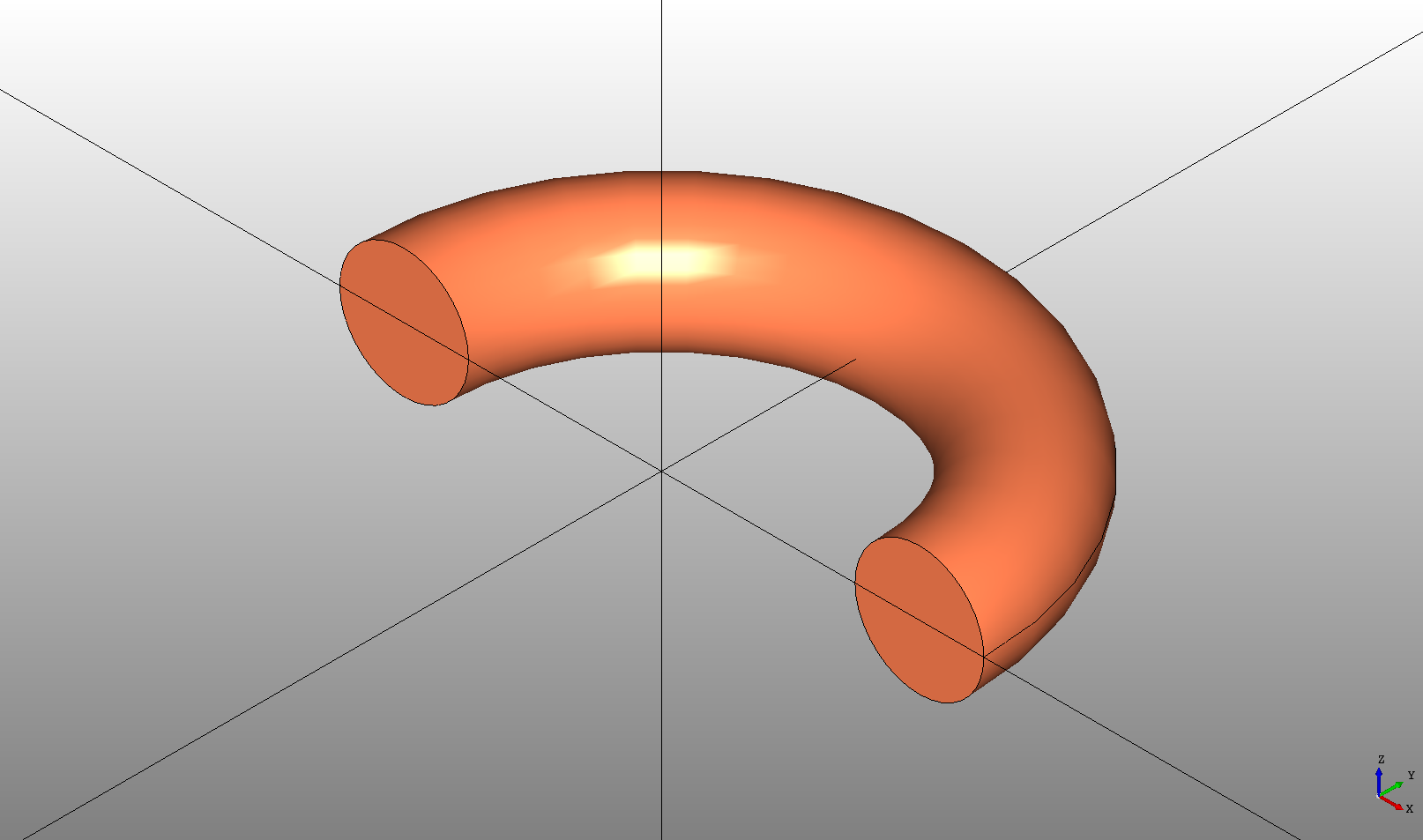And the start and stop angles of the circle profile can be limited by `angle2` and `angle3`

``````# Created in DeclaraCAD
from math import pi